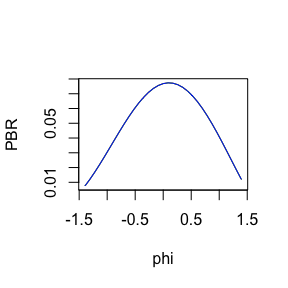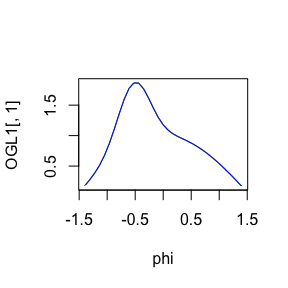# Introduction

## Rendering in R

The rendering by rgl within R is done using OpenGL 1.x (OGL1) code written largely by Daniel Adler in C++. OGL1 versions were released in the late 1990s, and rgl in the early 2000s. It’s unlikely that this will ever be updated to a more modern system: I’d prefer to move away from doing any rendering at all, and leave that to browsers that can display WebGL.

Rendering by rgl in web pages is done using WebGL 1 in Javascript code written by me. This is likely to be updated in the future, but for now the goal is to keep things simple, so compatibility with OGL1 is needed. I wrote code to generate shaders that automatically interpret things similar to the way OGL1 does it. There are facilities to support user-defined shaders, and they could use much more modern code; it’s also possible that the whole Javascript system will be updated to use a modern Javascript library rather than working with WebGL 1 forever.

## Rendering assumed by glTF

The glTF 2.0 format is much newer, with its first release in 2017. It assumes shaders are available that can interpret parameters described in terms of physically based rendering (PBR). This is a much more realistic system than OGL1 rendering. It aims for photo-realism, rather than the diagram-style rendering targeted by rgl.

## What is this document about?

The rgl2gltf package is intended to allow rgl scenes to be saved to files using glTF 2.0 formats, i.e. .gltf or .glb files, and objects from those files to be incorporated into rgl displays. Since those files assume PBR, we need to work out how to approximate the PBR appearance in OGL1 rendering and vice versa.

# Detailed calculations

## OGL1 rendering in rgl

The appearance of objects in rgl is controlled by some of the properties controlled by rgl::material3d(). These are the default settings:

library(rgl)
material3d()
#> $color #>  "#000000" #> #>$alpha
#>  1
#>
#> $lit #>  TRUE #> #>$ambient
#>  "#000000"
#>
#> $specular #>  "#FFFFFF" #> #>$emission
#>  "#000000"
#>
#> $shininess #>  50 #> #>$smooth
#>  TRUE
#>
#> $front #>  "filled" #> #>$back
#>  "filled"
#>
#> $size #>  3 #> #>$lwd
#>  1
#>
#> $fog #>  TRUE #> #>$point_antialias
#>  FALSE
#>
#> $line_antialias #>  FALSE #> #>$texture
#> NULL
#>
#> $textype #>  "rgb" #> #>$texmipmap
#>  FALSE
#>
#> $texminfilter #>  "linear" #> #>$texmagfilter
#>  "linear"
#>
#> $texenvmap #>  FALSE #> #>$depth_mask
#>  TRUE
#>
#> $depth_test #>  "less" #> #>$isTransparent
#>  FALSE
#>
#> $polygon_offset #>  0 0 #> #>$margin
#>  ""
#>
#> $floating #>  FALSE #> #>$tag
#>  ""
#>
#> \$blend
#>  "src_alpha"           "one_minus_src_alpha"

Colors are assumed to be RGB with 8 bits per channel defined using R color notation, for example "#FF0000" is pure red. alpha controls transparency, running from 0 to 1: 0 is fully transparent (invisible), 1 is fully opaque. If lit is FALSE, that’s it: the color is displayed without any modification.

When lit = TRUE the shading algorithm comes into play. The material properties ambient, specular and emission are additional colors, and the shininess, smooth and fog parameters come into the calculation. There’s a possibility to have a single texture: an image file whose characteristics can be used to modify the color or alpha channel, with a location in the image defined by “texture coordinates” associated with the surface being rendered.

The above parameters are combined with global properties of the lighting: it has a direction as well as ambient, diffuse and specular colors.

Consider the following image:

xyz <- cbind(c(0, 1, 1, 0), c(0, 0, 1, 1), c(0, 0, 1, 1))
st <- xyz[, 1:2]
texcoords = st,
texture = system.file("textures/rgl2.png", package = "rgl"),
color = "steelblue")

This is drawn using these two automatically generated shaders. The vertex shader controls the positioning of the quadrilateral. It is called for each vertex of the quad, and controls the position where they are displayed.

  /* ****** quads object 13 vertex shader ****** */
#ifdef GL_ES
#ifdef GL_FRAGMENT_PRECISION_HIGH
precision highp float;
#else
precision mediump float;
#endif
#endif

/* these are constants for all vertices */
uniform mat4 mvMatrix;
uniform mat4 prMatrix;
uniform mat4 normMatrix;

/* these are properties associated with each vertex */
attribute vec3 aPos;
attribute vec4 aCol;
attribute vec3 aNorm;
attribute vec2 aTexcoord;

/* These are computed and passed to the fragment shader */
varying vec4 vCol;
varying vec4 vPosition;
varying vec4 vNormal;
varying vec2 vTexcoord;

void main(void) {
vPosition = mvMatrix * vec4(aPos, 1.);
gl_Position = prMatrix * vPosition;
vCol = aCol;
vNormal = normMatrix * vec4(-aNorm, dot(aNorm, aPos));
vTexcoord = aTexcoord;
}

The fragment shader is called for each pixel on the surface of the quad, and controls the appearance. I’ve improved the formatting a bit and added extra comments:

/* ****** quads object 13 fragment shader ****** */
#ifdef GL_ES
#ifdef GL_FRAGMENT_PRECISION_HIGH
precision highp float;
#else
precision mediump float;
#endif
#endif

/* these variables are interpolated
between the values calculated for each vertex */

varying vec4 vCol; // carries alpha
varying vec4 vPosition;
varying vec2 vTexcoord;
varying vec4 vNormal;

/* these are constant for all fragments */
uniform sampler2D uSampler;
uniform int uFogMode;
uniform vec3 uFogColor;
uniform vec4 uFogParms;
uniform mat4 mvMatrix;
uniform vec3 emission;
uniform float shininess;
uniform vec3 ambient0;
uniform vec3 specular0; // light*material
uniform vec3 diffuse0;
uniform vec3 lightDir0;
uniform bool viewpoint0;
uniform bool finite0;

void main(void) {

/* these are local variables */

vec4 fragColor;
vec3 n = normalize(vNormal.xyz);
vec3 eye = normalize(-vPosition.xyz);
vec3 lightdir;
vec4 colDiff;
vec3 halfVec;
vec4 lighteffect = vec4(emission, 0.);
vec3 col;
float nDotL;

n = -faceforward(n, n, eye);
colDiff = vec4(vCol.rgb * diffuse0, vCol.a);
lightdir = lightDir0;
if (!viewpoint0)
lightdir = (mvMatrix * vec4(lightdir, 1.)).xyz;
if (!finite0) {
halfVec = normalize(lightdir + eye);
} else {
lightdir = normalize(lightdir - vPosition.xyz);
halfVec = normalize(lightdir + eye);
}
col = ambient0;
nDotL = dot(n, lightdir);
col = col + max(nDotL, 0.) * colDiff.rgb;
col = col + pow(max(dot(halfVec, n), 0.), shininess) * specular0;
lighteffect = lighteffect + vec4(col, colDiff.a);
vec4 textureColor = lighteffect*vec4(texture2D(uSampler, vTexcoord).rgb, 1.);
fragColor = textureColor;
float fogF;
if (uFogMode > 0) {
fogF = (uFogParms.y - vPosition.z/vPosition.w)/(uFogParms.y - uFogParms.x);
if (uFogMode > 1)
fogF = mix(uFogParms.w, 1.0, fogF);
fogF = fogF*uFogParms.z;
if (uFogMode == 2)
fogF = 1.0 - exp(-fogF);
else if (uFogMode == 3)
fogF = 1.0 - exp(-fogF*fogF);
fogF = clamp(fogF, 0.0, 1.0);
gl_FragColor = vec4(mix(fragColor.rgb, uFogColor, fogF), fragColor.a);
} else gl_FragColor = fragColor;
}

I’ll use the same notation as https://registry.khronos.org/glTF/specs/2.0/glTF-2.0.html#implementation, plus some more:

• $$V$$ is the normalized vector from the shading location to the eye
• $$L$$ is the normalized vector from the shading location to the light
• $$N$$ is the surface normal in the same space as the above values
• $$H$$ is the half vector, where $$H = normalize(L + V)$$
• $$\chi^+(x)$$ is the Heaviside function max(x, 0)
• $$s$$ is the shininess
• $$C_a$$, $$C_d$$, $$C_s$$ and $$C_e$$ are the material colors, ambient, diffuse (just color in the material), specular and emission respectively.
• $$L_a$$, $$L_d$$ and $$L_s$$ are the ambient, diffuse and specular light values respectively.

Ignoring fog and texture for now, the fragment shader implements the following computation of the color at a particular location: $C_e + C_aL_a + \chi^+(N \cdot L) C_d L_d + \chi^+(H \cdot N)^s C_sL_s$ The texture multiplies this value by the texture color at that location, and fog mixes it with the fog color in a proportion determined by the fogMode parameter and the depth in the scene.

## PBR rendering in glTF

The glTF specification presents a sample implementation, with several comments following to describe possible improvements. The simple computation introduces some new notation based on the material properties in glTF:

• $$\alpha$$ is the square of the roughness value
• $$m$$ is the metallic value between 0 and 1
• $$\textit{lerp}(a, b, t)$$ is the linear interpolation function returning $$(1-t)a + tb$$, aka $$\textit{mix}(a, b, t)$$.

Using this notation and the previous notation, it computes the “bidirectional reflectance distribution function” or BRDF as $D(\alpha) := \frac{1}{\pi}\frac{\alpha^2 \chi^+(N \cdot H)}{[(N \cdot H)^2 (\alpha^2 - 1) + 1]^2} \\ G(\alpha) := \frac{2 | N \cdot L | \chi^+(H \cdot L)}{|N\cdot L| + \sqrt{\alpha^2 + (1-\alpha^2)(N \cdot L)^2}}\frac{2|N\cdot V|\chi^+(H \cdot V)}{|N \cdot V| + \sqrt{\alpha^2 + (1-\alpha^2)(N \cdot V)^2}}\\ \textit{f0} \leftarrow \textit{lerp}(0.04, C_d, m) = 0.04(1-m) + mC_d \\ F \leftarrow \textit{f0} + (1 - \textit{f0})(1- |V \cdot H|)^5 \\ \textit{f_diffuse} \leftarrow \frac{1}{\pi}(1-F)\textit{lerp}(C_d, 0, m) = \frac{1}{\pi}(1-F)(1-m)C_d\\ \textit{f_specular} \leftarrow \frac{F D(\alpha) G(\alpha)}{4 |V\cdot N| |L \cdot N|}$ These values are combined into a single value f_diffuse + f_specular which is multiplied by cosine of the incoming light angle ($$N \cdot L$$ in the notation above) and used in ray-tracing based rendering.

## Assumptions in the approximations

The default light in rgl has all of $$L_a$$, $$L_d$$ and $$L_s$$ being white, i.e. 1. Most materials have $$C_a$$ and $$C_e$$ set to black, i.e. 0. We can thus ignore ambient and emission contributions, or assume they add to the diffuse and specular contributions from the formula. With that assumption, the output from rgl simplifies to $\chi^+(N \cdot L) C_d + \chi^+(H \cdot N)^s C_s$

We’ll also assume that $$C_d$$ is fixed as color in rgl and as baseColor in PBR. Then matching given PBR parameters in OGL1 terms comes down to using them to choose shininess $$s$$ and specular color $$C_s$$ so that the expression above, which defines a function depending on $$N$$, $$L$$ and $$V$$, approximates the BRDF calculation which defines a different function depending on those three vectors.

We start by rotating the coordinate system so that $$N = (0,0,1)$$ and $$V = (\sin \theta, 0, \cos \theta)$$, where $$\theta$$ is the angle between $$V$$ and $$N$$, and $$V \cdot N = \cos \theta$$.

What we’d like to do is to match the appearance over the whole range of $$\theta$$ and $$L$$, but that seems hard, so instead we’ll try a few fixed values of $$\theta$$ and try to match graphs of BRDF and OGL1.

Let’s try it:


# Typical PRB parameters
roughness <- 1
metalness <- 1
baseColor <- c(1, 1, 1)

# Default rgl parameters
s <- 50
Cs <- c(1,1,1)

Cd <- baseColor
m <- metalness
alpha <- roughness^2

h <- c(0.999, 0.5)

N <- c(0,0,1)
theta <- 30 * pi/180 # angle between V and N
V <- c(sin(theta), 0, cos(theta))
VdotN <- sum(V*N)

phi <- seq(-80, 80, by = 5)*pi/180 # angle between L and N
PBR <- OGL1 <- matrix(NA, nrow=length(phi), ncol=3)
for (j in seq_along(phi)) {
L <- c(sin(phi[j]), 0, cos(phi[j]))
H <- (L + V)/sqrt(sum((L + V)^2))
HdotN <- sum(H*N)
HdotL <- sum(H*L)
NdotL <- sum(N*L)
HdotV <- sum(H*V)
D <- (1/pi)*alpha^2*max(0, HdotN)/(HdotN^2 * (alpha^2 - 1) + 1)^2
G <- 2*NdotL*max(HdotL, 0)*2*VdotN*max(HdotV, 0)/
(NdotL + sqrt(alpha^2+ (1-alpha^2)*NdotL^2))/
(VdotN + sqrt(alpha^2+ (1-alpha^2)*VdotN^2))
f0 <- 0.04*(1-m) + m*Cd
F <- f0 + (1 - f0)*(1 - HdotV)^5
f_diffuse <- (1/pi)*(1-F)*(1-m)*Cd
f_specular <- F*D*G/4/abs(VdotN)/abs(NdotL)
PBR[j,] <- (f_diffuse + f_specular)*NdotL
OGL1[j,] <- max(NdotL, 0)*Cd + max(HdotN, 0)^s*Cs
}
plot(phi, PBR[,1], type="l", col = "red", ylim=range(PBR), ylab="PBR")
lines(phi, PBR[,2], col = "green")
lines(phi, PBR[,3], col = "blue")plot(phi, OGL1[,1], type="l", col = "red", ylim=range(OGL1))
lines(phi, OGL1[,2], col = "green")
lines(phi, OGL1[,3], col = "blue")This looks pretty hopeless.